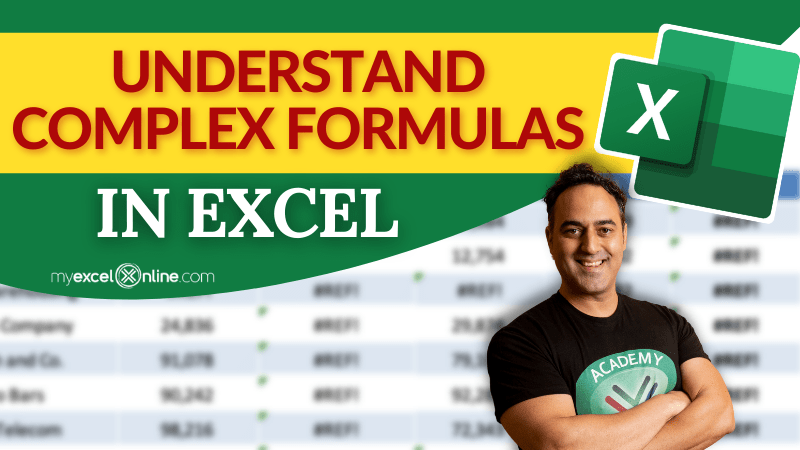Excel has bombarded its users with a plethora of functions and formulas that can help solve any person’s problems and speed things up.

Having too many formulas gives rise to the problem of understanding the inner workings of formulas. It can be challenging to understand how complex formulas in Excel works, what to enter in each argument, and what is the step-by-step process of the formula.

In this article, we will cover how to write a complex formula using an Argument Wizard and how to understand the workings of a formula using Evaluate Formula.

So, without further ado. Let us dive in! Download this Excel workbook so you can practice along with us:

Arguments wizard

Sometimes, you may not be sure how a specific formula works or what will be the syntax of that formula. The best way of understanding these requirements is through the formula arguments wizard.

To better understand this, let us take the example using the VLOOKUP formula. Here, we need to extract the salary of Rachael in cell D15.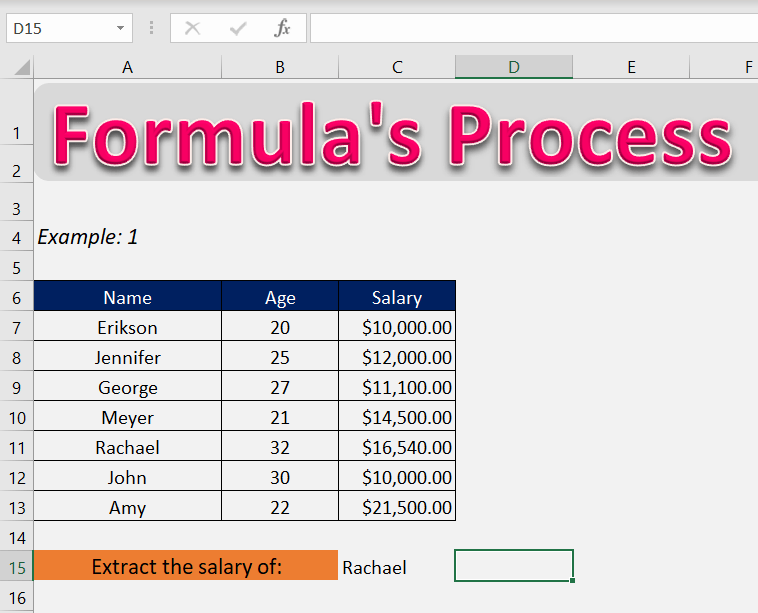Let’s open the arguments wizard for the VLOOKUP formula.

STEP 1: Enter the VLOOKUP function: =VLOOKUP(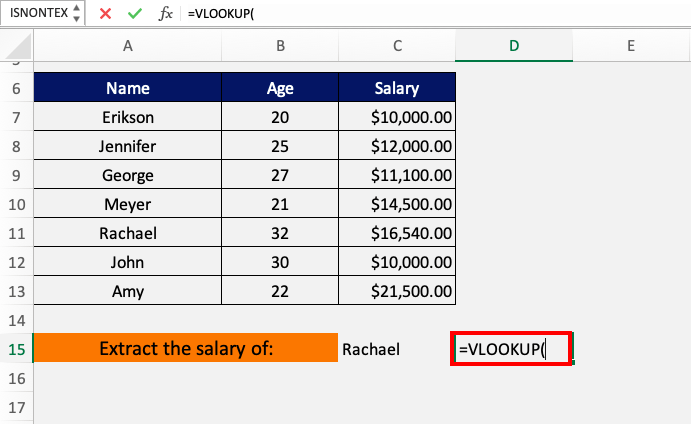STEP 2: Press Ctrl+A or click on the fx button in the formula bar to open the arguments wizard window.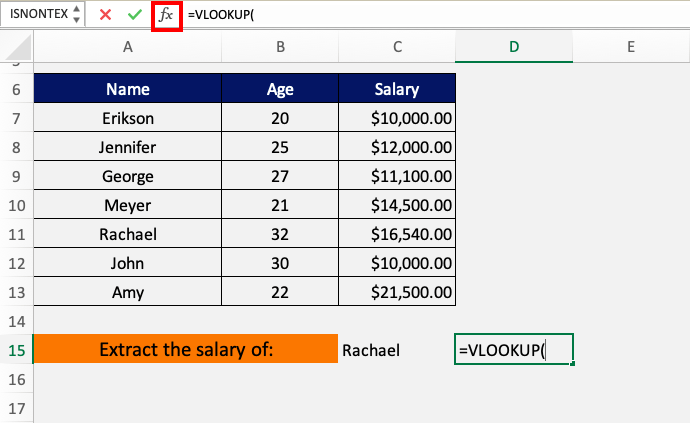This is how the formula wizard looks like.

It shows all the arguments in sequential order. It then explains the use of the function and the details of the argument selected.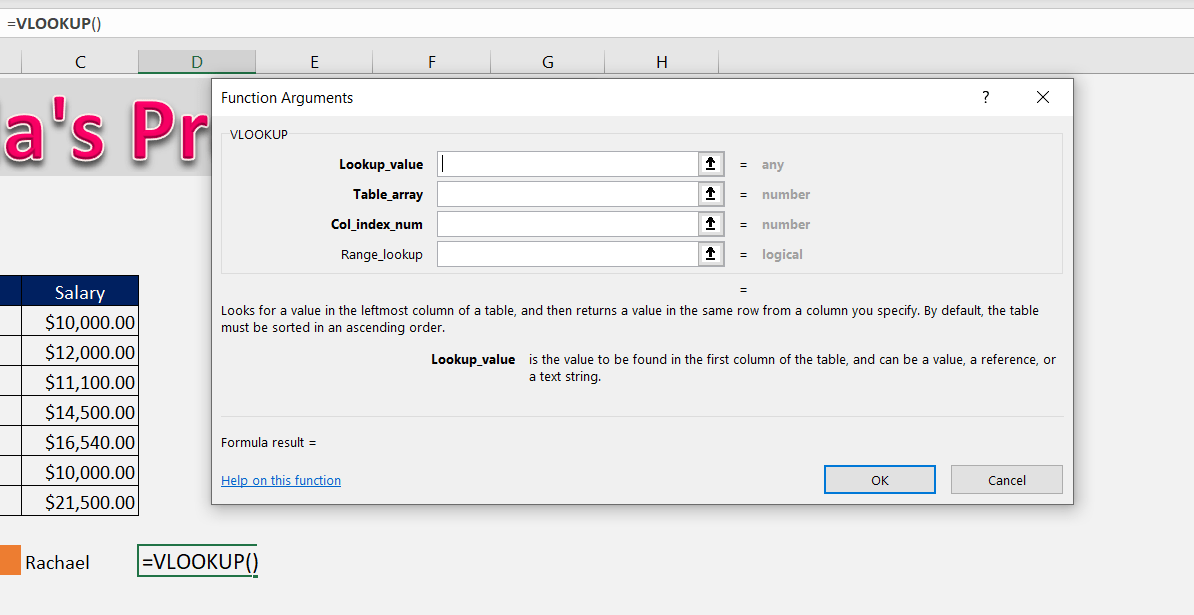STEP 3: Select the first argument i.e. Lookup_value. Here, cell C15 contains the name, Rachael.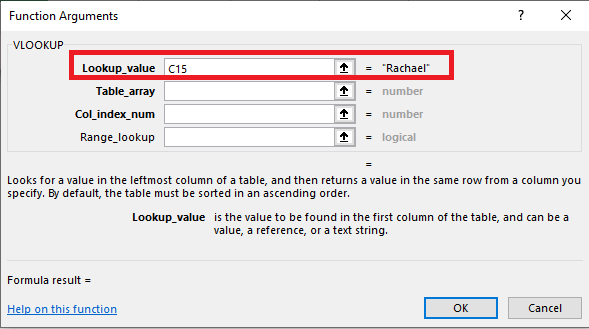You can also see the description of the first argument is written at the bottom of the dialog box.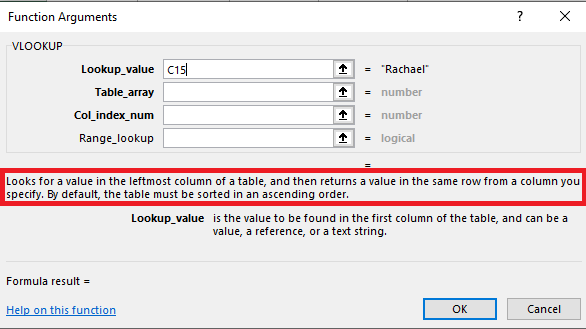STEP 4: Select the second argument i.e. Table_array and select the table range from where you can search i.e. A7:C13.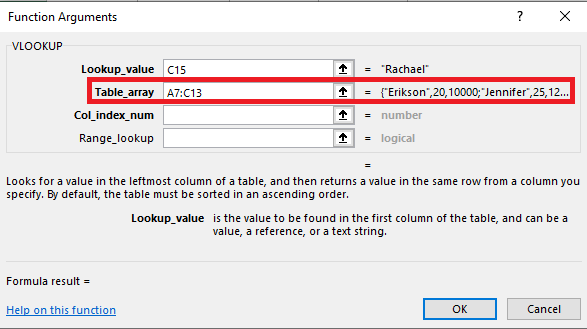STEP 5: Select the third argument i.e. col_index_num and enter the column number from which to extract the data. In this example, it should be 3.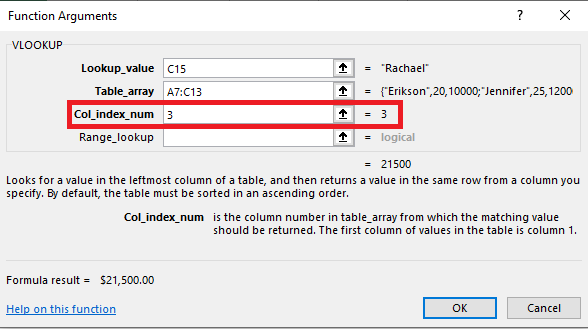STEP 6: Lastly, select the fourth argument i.e. range_lookup. Since we want an exact match type FALSE or 0.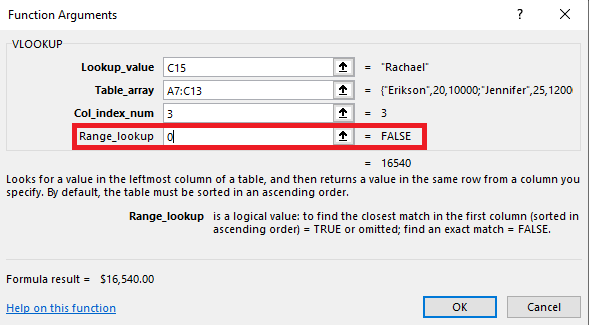After entering the details, the wizard would look like below. It would even show a preview of the argument values and the answer below it if the inputs were correct.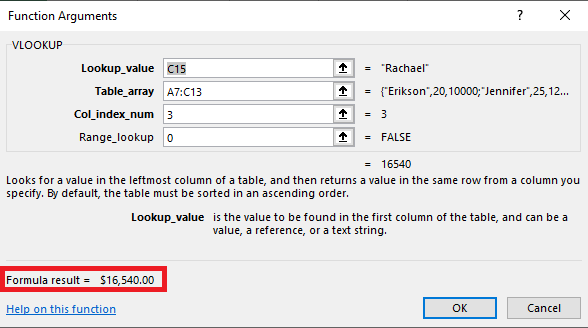STEP 7: Press OK.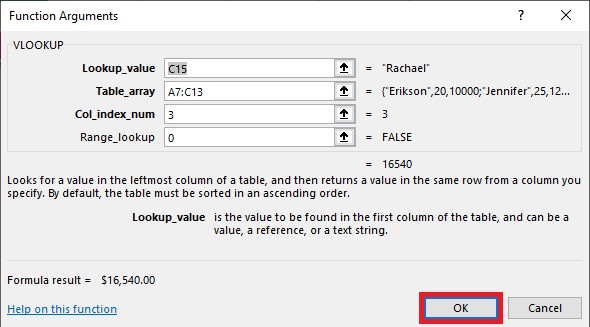The formula will be entered in cell D15 and it will show the result, i.e., the salary of Rachael – \$16,540.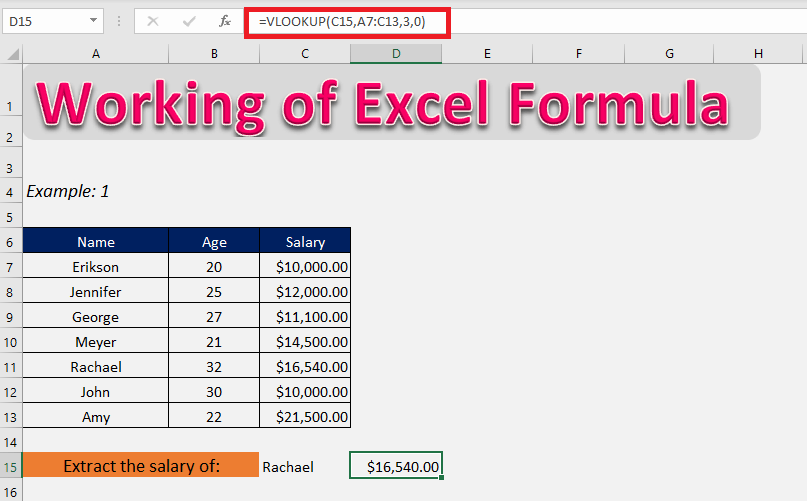Evaluate Formula

We can even use evaluate formula feature in Excel to understand how complex formulas in Excel works step by step and provides the final result.

It solves the problem that we face countless times wherein we have a hard time understanding formulas. It is useful in mastering how a formula works and it is also a great tool for debugging.

Let’s example look at the formula below. Here, we want to check if the average salary is greater than or equal to the standard set i.e. \$12,000.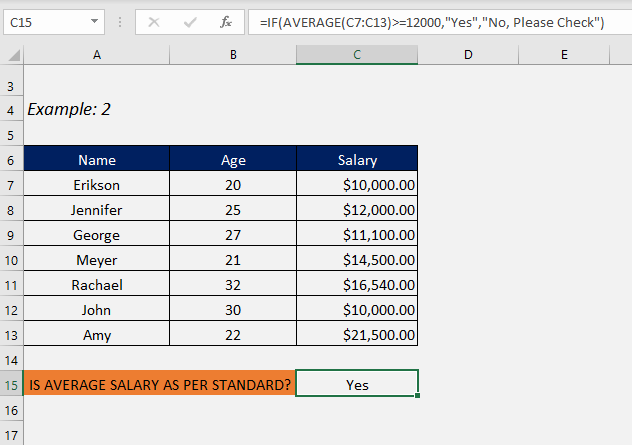Now, let us use the evaluate formula feature to see the workings of complex formulas in Excel!

STEP 1: Select the cell (i.e. C15) that contains the formula you want to evaluate.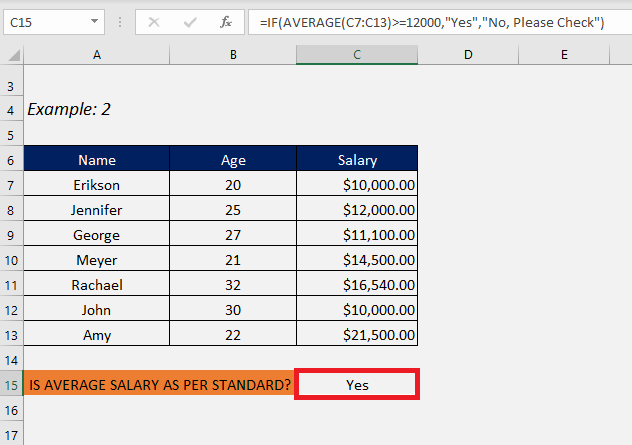STEP 2: Go to Formulas > Evaluate Formula.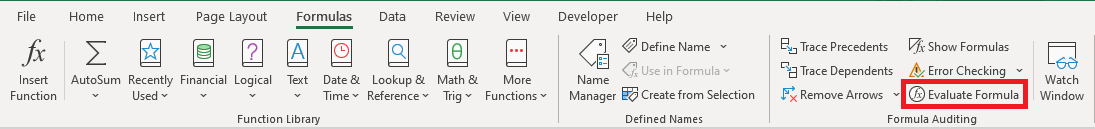STEP 3: The formula will now be displayed in the Evaluate Formula dialog box and the part of the Excel complex formula that will be evaluated first is underlined.

Click Evaluate.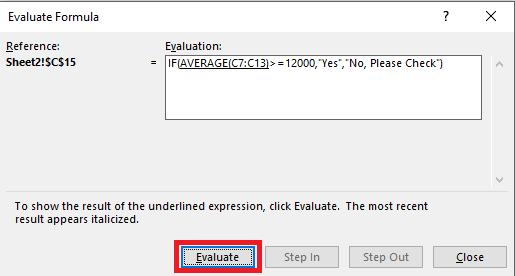STEP 4: The average will be calculated and displayed as 13662.8514….

Click Evaluate.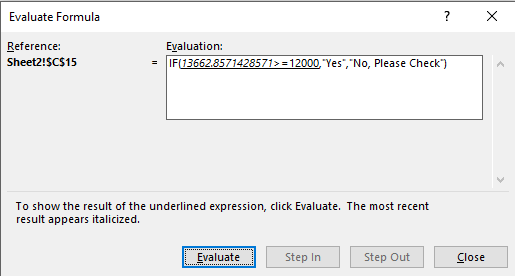STEP 5: Since the average is greater than 12000 and the logical condition of the IF formula is met, it will display TRUE.

Click Evaluate.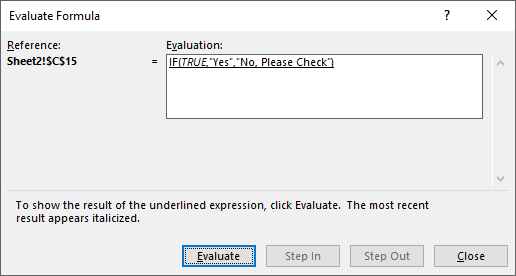STEP 6: Lastly, the text if the condition is met is Yes. So yes is displayed.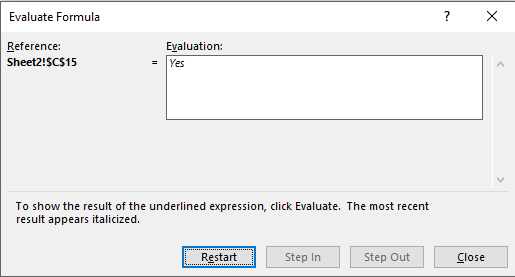You can click either Restart to see the step-by-step evaluation again or Close to close the dialog box.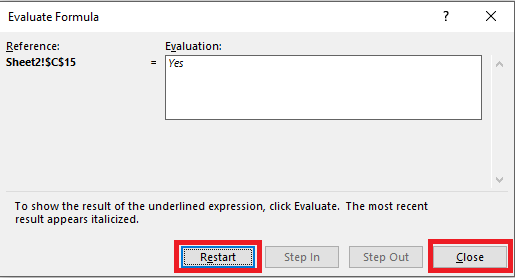### Conclusion

Now you can easily open the function arguments wizard to understand the requirements of the complex formulas in Excel and each of its arguments. This will help you work with newer functions and improve your operations with functions you are already familiar with.

Also, you can now use evaluate formula to see how a formula gave us the result in a few easy steps!

We hope this tutorial helped you to understand and learn Excel formulas better and aid you in smooth functioning on Excel.